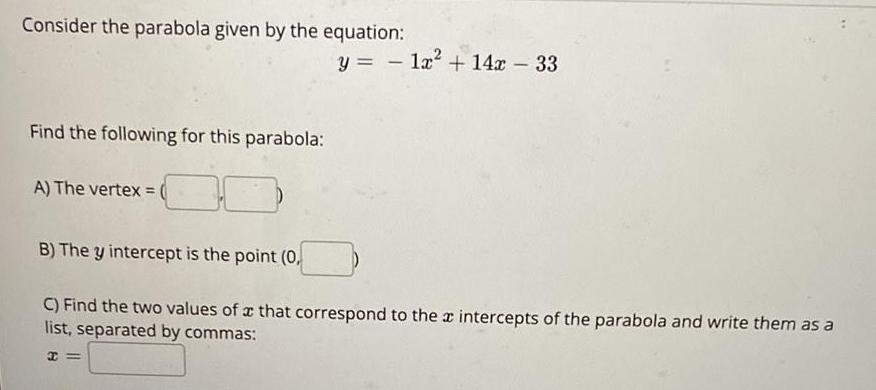Question:

# Consider the parabola given by the equation: y = 1x² + 14x

Last updated: 8/14/2022Consider the parabola given by the equation: y = 1x² + 14x - 33 Find the following for this parabola: A) The vertex = ( B) The y intercept is the point (0, C) Find the two values of a that correspond to the intercepts of the parabola and write them as a list, separated by commas: